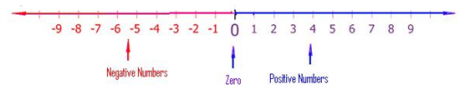### Multiplication and Division of Directed Numbers

Mathematics J.S.S 2 First Term

Theme: Numbers & Numeration

Sub Theme: Whole Number

WEEK 10

Multiplication and Division of Directed Numbers

Performance Objectives

Students should be able to;

1. Carry out multiplications  involving direct numbers
2. Carry out Divisions involving direct numbers

Addition and Subtraction of Directed Number Line (Revision)

Content

Directed numbers are positive or negative numbers.

Numbers can be shown on a number line which extends above and below zero. This gives positive and negative numbers.The signs + and – show the direction from 0. Positive and negative numbers are called directed numbers.

Addition: To add using the number line, we move to the right (forward) of the number line counting each step till the addition is complete. The number at.... View More